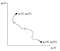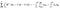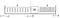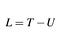# Lagrangians

Larmor had an intense, almost mystical devotion to the principle of least action…To [him] it was the ultimate natural principle — the mainspring of the Universe.~Arthur Eddington, quoted in Zangwill (2013)¹.

Two beads with masses M₁ and M₂ slide without friction on a ring of radius R. The masses are connected over the minor arc A by a spring with spring constant k₁ and natural length a and over the major arc B by a spring with constant k₂ and natural length a₂. The ring lies flat on a table.

Suppose that we are asked to find the equations of motion for the beads. In principle, Newton’s laws of motion are enough to solve this problem. But in practice, finding equations of motion with Newton’s laws for a complicated system is tedious and difficult. And even this foreboding problem pales in comparison to the complexity of the mechanical systems that actually occur in the real world.

When we find a problem to be too difficult, a good way to proceed is to try to change our perspective on the problem. But what kind of new perspective should we adopt?

A surprising answer comes to us from the study of optics.

## Fermat’s principle of least time

The basic laws of reflection of light rays have been known since antiquity. When a ray of light is incident upon a reflecting surface, then the angle of incidence is equal to the angle of reflection:

Hero of Alexandria (circa 10 CE to 70 CE) was able to prove from this fact that the path taken by the ray of light is the path of shortest length that it could possibly take between the source, the mirror, and its destination. That is to say, given points A and C, the ray of light reflects off of the mirror at a point B such that the length of the path is minimal. He also invented the first vending machine, which is neat.

What the ancients could not resolve was the problem of refraction. When a ray of light is incident on a surface that separates two different materials (the canonical example is air and water), the ray of light bends.

A ray of light that leaves point A, strikes the surface of the water at point B, and ultimately arrives at point C does not take the shortest possible path between points A and C, which would be a straight line.

The law that relates the angles of incidence and refraction is known as Snell’s Law, after Willebrord Snellius (1580–1626), although it was first discovered by Ibn Sahl in 984 CE. It states that:

Where n is the index of refraction of each medium. This law was discovered experimentally and lacked a theoretical justification. It was Pierre de Fermat (1607–1665) who provided one. Expanding on the ancient Greeks’ discovery that reflecting light takes the path of shortest length between two points, Fermat proposed that instead light (which by then was widely thought to have finite speed) took the path between two points that took the shortest amount of time. From this postulate, he was able to derive Snell’s Law. This is a common exercise in intermediate physics textbooks and I may post it in a short follow-up article to this one.

Fermat’s Principle is considered to be the first historical example of an important physical idea: Nature will seek to carry out physical processes in such a way as to minimize some important quantities characteristic of the process. The somewhat mystical language employed here is an historical artifact: the idea was first conceived by Maupertuis (1698–1759), who constructed the idea on purely theological grounds. Of course, there is nothing at all supernatural happening here, and this idea was put on a firm scientific footing in the 19th century. For the remainder of this article we will explore this idea by developing the theory of the Langrangian formalism.

## Constraints , generalized coordinates, and configuration space

We can always express the state of a system in terms of the Cartesian coordinates (x,y,z) of its constituent particles, but depending on the problem we may be able to pick more useful variables. If we do this strategically, we can even reduce the number of variables that we need to account for. For example, a point P on the surface of a sphere can be completely specified by the polar and azimuthal angles (θ,φ), as in the following diagram:

The arbitrary variables q₁, q₂, …, qₙ are called generalized coordinates. They are generalized in the sense that they can be whatever we want as long as they completely specify the state of the system and none of them have any functional dependence on each other. The time derivatives of the generalized coordinates are called the generalized velocities. Knowing which variables to use is an intuitive skill that can only be learned through practice.

When we have picked our generalized coordinates, we are able to represent the state of a system at any time as a point in configuration space. The path traced by the system in configuration space as it evolves through time is called the trajectory.A trajectory for the time evolution of a system with two degrees of freedom.

So the problem of determining the evolution of a mechanical system through time can be approached by asking which trajectory the system follows in configuration space.

## Interlude: Functionals

Suppose that as a ray of light travels between points A and B it follows a path in the plane given by (x,f(x)):

Let v(x) be the speed of light as a function of x. In a medium with index of refraction n, v=c/n. In this problem, n is a function of y, with:

The y coordinate of the path becomes negative when the x coordinate becomes positive, so we can write:

The differential element of a curve (x,y=f(x)) has length:

By the definition of velocity v=ds/dt, so we can represent the total travel time of the ray as an integral:

This definite integral takes a function f(x) and returns a number T, in a sense, it’s a function that takes a function rather than a variable as its input. Such objects are called functionals. We can interpret Fermat’s principle of least time as saying that the path (x,f(x)) has f(x) such that the time functional T[f(x)] has a minimum value.

Just as we can define the derivative of a function f(x) with respect to the variable x as:

We can define the functional derivative of a functional δJ[f(x)]/δf(x), which gives the rate of change of J as f(x) is replaced by f(x)+η(x), by the relation:

The function η is called the variation of f(x), which is an arbitrary function that vanishes on the boundary of the domain of integration. In this article, we are going to be interested in functionals of the form:

Here, F is a function that’s been composed with x, f(x), and f’(x). Let’s obtain the functional derivative of J using the definition.

Line 3 is by the chain rule. Line 5 is because f_ε=f for ε=0, and line 6 is from using integration by parts on the second term in the integrand of part 5. Since η vanishes at a and b, this means that by cancelling the integral we get:

This also applies if J is a functional of more functions besides f(x) as long as only f(x) is varied. To see why, let f(x) = (f₁(x), f₂(x), …, fₙ(x)) and vary only fₐ. Then J takes the following form:

So only fₐ will survive when we get to the chain rule step in line (3) above.

## Functionals of the trajectory

Let q(t)=(q₁, q₂, …, qₙ) be the position vector of the trajectory in configuration space. To analyze the trajectory from a functional point of view, we need to come up with some functionals that depend on the entire state of the system.

The potential energy function U depends on the entire state, and if we assume that the system is conservative (which we will from now on) then U does not depend on the generalized velocities. A simple functional that we could use is the average of U over the trajectory:

The functional ⟨U⟩ has the right form to be applicable to the result of the last section so:

Since U does not depend on the velocities, the rightmost partial derivative vanishes (although we will need this form later) and we can write:

Since we are dealing with a conservative system, we are free to define the generalized force:

Therefore:

The functional ⟨T⟩ also has the right form for the formula from the last section to apply:

Now that we have the functional derivatives in a workable form, we can move on to the actual physics.

## The Lagrangian

A conservative system can be said to evolve through time by exchanging kinetic and potential energy. This happens because a conservative force acts on the system to change its potential energy into kinetic energy, or vice versa.

Suppose that along the trajectory the average potential energy is ⟨U⟩ and that after a variation of the trajectory the average potential energy changes into ⟨U⟩+∆U. The variation of the trajectory has therefore made some additional energy available to contribute to the average kinetic energy of the system. We might guess that the change in the average kinetic energy is equal to the change in the average potential energy. We will now prove that this is the case when any coordinate qₐ is varied to qₐ+δqₐ. That is, I claim that:

If the system is composed of N particles, then the kinetic energy of the ith particle is:

On applying the product rule for the derivative of the dot product, we get:

By the chain rule:

So:

Now we consider the derivative of T with respect to the generalized velocity. Following the same reasoning as above:

The time derivative of this quantity is:

By summing over all N particles, we find that:

Where the F with superscript S denotes the ordinary Newtonian force on the ith particle as a function of the generalized coordinates. I will now complete the proof by showing that this sum is equal to the generalized force Fₐ.

Let A and B be two “locations” in configuration space connected by a straight line parallel to the qₐ axis. For the case of two degrees of freedom, this might look like:

The line integral from A to B of this quantity is

The line integral on the right is over each path C_i taken by the position vector of the ith particle as qₐ goes from A to B. By definition, this is the negative of the difference in potential energy of the system between A and B:The middle equality is due to the Fundamental Theorem of Calculus.

So therefore:

And this lets us complete the proof:

This means that with respect to variations of the true trajectory, we have:

This integral is called the action functional. What we have done is proven Hamilton’s Principle of Least Action: The trajectory of a mechanical system in configuration space is that which minimizes the action functional (technically it can also be a maximum, but this is very rare and doesn’t change anything).

The function L=T-U is so important that it has its own name. It is called the Lagrangian.We now obtain the Euler-Lagrange equation:

The Euler-Lagrange equation gives the equation of motion for each of the qₐ. This equation is the payoff for all of our work. For many important mechanical systems, once you have the Lagrangian, finding the equations of motion is just plug-and-chug.

We can say that all of the information about the evolution through time of the state of a standard system is contained in the Lagrangian, and so the phrasing in the subtitle of this article is justified: a Lagrangian for the system can be said to constitute a theory of the system’s behavior.

## When is the Lagrangian approach valid?

Many important mechanical problems involved motion along a fixed surface or path. For example, if a particle moves on the surface of a sphere then its position coordinates (x,y,z) satisfy x²+y²+z²-R²=0. Constraints which can be expressed in the form f(x,y,z)=0 are called geometric constraints.

We may also have a kinematic constraint. As geometric constrains place a restriction on the position of a particle, a kinematic constraint puts a restriction on the velocity of a particle. For example, if we say that a particle’s velocity is entirely in the x-direction, then this is a kinematic constraint which says that the y and z components of the velocity are zero. After integrating, this kinematic constraint becomes a geometric constraint which says that the y and z components of the position are constant. When this is possible, we say that the kinematic constraint is integrable.

The Lagrangian formalism applies only to systems with exclusively geometric and integrable kinematic constraints. We call such a system holonomic. An important category of non-holonomic constraints are those involving inequalities. For example, if a ball is on a table and tied to the origin by a string of length l, then the constraint is x²+y²≤l².

Lagrangian mechanics is always valid for conservative forces. Sometimes it can be extended to non-conservative forces, but this isn’t always a good approach.

While that may sound like a weakness, it turns out that the class of conservative, holonomic systems is very large and contains most of the interesting problems that one is likely to encounter.

So with all of that said, let’s now go through some examples to see the Lagrangian formalism in action.

## A pendulum

A mass m is attached to the end of a rigid, light rod of length l,and makes small-angle oscillations.

While the motion is in two dimensions, the symmetry of the problem suggests that the motion can be specified completely by the angle θ.

It’s often a good idea to start in Cartesian coordinates and then convert. The kinetic energy is, as always:

The potential due to gravity U=mgy. Therefore:

Then we use x=lsinθ, y = lcosθ to find L in terms of the generalized coordinate θ by computing x-dot and y-dot with the chain rule:

Then Lagrange’s equation of motion for θ is:

## A block between two springs

A block of mass M moves back and forth in one direction. The block is attached to two identical springs of spring constant k and natural length a. The springs are fixed to the walls x=±a.Note: Ignore the extent of the block and treat it as a point mass.

The left spring is stretched when x is positive and compressed if x is negative, so the length of the left spring is a+x. The right spring is stretched when x is negative and compressed if x is positive, so its length is a-x. Therefore the kinetic and potential energies are:

So the Lagrangian is:

Now we just plug this in to the Euler-Lagrange equation for x:

## Two beads on a ring

We are now finally ready to solve the problem proposed in the opening paragraph.

Let U₁ and U₂ be the potentials of the springs covering minor arc A and major arc B, respectively. The arc lengths of A and B are (θ₂-θ₁)R and (2π+θ₁-θ₂)R. So the potentials for the two springs are:

The kinetic energy for a particle of mass m and angular velocity ω revolving at fixed distance R from the origin is ½mR²ω², so the kinetic energies are:

Since L=T-U, the Lagrangian is:

To find the equations of motion all we have to do is plug this in to the Euler-Lagrange equations for θ₁ and θ₂:

So the equations of motion are:

Obtaining these equations with the Lagrangian method was far easier than it would have been if we had tried to use Newton’s laws.

## Conclusion: What does all of this mean?

What have we actually done here, and why go to all the trouble?

It’s important to understand that we have not introduced any new physical theories. All of the underlying physics and the rules about forces, energy, and so on have remained unchanged. What we did change was our perspective. We took those rules and looked at them in a different way, and so we developed a new understanding of what those rules are and what we can do with them.

As for why we went to all the trouble, the most direct answer is that we wanted something that was easier to work with than Newton’s laws for analyzing certain physical systems. But that’s not the only reason. Newton’s laws can in principle solve any problem in classical mechanics, but they are tremendously ungainly for analyzing classical fields and utterly meaningless in quantum physics. On the other hand, the Lagrangian formalism, and Hamilton’s Principle more generally, can be adapted to quantum physics. We will see how in an upcoming article.

As always, thanks for reading. If there are any mistakes or if you want clarification on anything, feel free to let me know in the comments.

## Correction (Feb 20, 2019)

When this article was first published it incorrectly stated that the definition of the functional derivative was:

In which δD is the Dirac delta distribution. This is not in fact the definition but rather a mnemonic that is used in physics textbooks. With the correct definition, the sections titled Interlude: Functionals and Functionals of the trajectory were able to be rewritten in a much more elegant form. Huge thanks to Reddit user u/localhorst in r/math for their patience and constructive criticism.

## Bibliography

1: Modern Electrodynamics by Andrew Zangwill (2013).

2: Quantum Field Theory for the Gifted Amateur by Tom Lancaster and Stephen Blundell (2014).I have to put this here so Medium will let me use it as the thumbnail.

Written by

## Dialogue & Discourse

#### News and ideas worthy of discourse. Fundamentally informative and intelligently analytical.

Welcome to a place where words matter. On Medium, smart voices and original ideas take center stage - with no ads in sight. Watch
Follow all the topics you care about, and we’ll deliver the best stories for you to your homepage and inbox. Explore
Get unlimited access to the best stories on Medium — and support writers while you’re at it. Just \$5/month. Upgrade